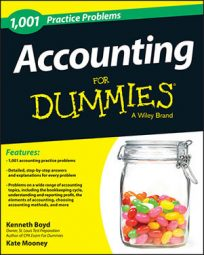##### AccountingAn accountant uses depreciation is to allocate the cost of a fixed asset over the years of its useful life. The straight-line depreciation method is the most popular type because it allocates the same amount of depreciation to each year the asset is in use.

The following practice questions show the straight-line depreciation method in action.

## Practice questions

1. A company purchases a machine for its manufacturing facility for \$90,000 in January and as of December has recorded only 11 months of depreciation. The machinery is estimated to have a useful life of 5 years. What is the proper entry to record the year-end adjustment for depreciation, assuming the straight-line method is used?

2. A company purchased a truck for \$25,000 on January 1 and as of December has not recorded any depreciation. The truck is estimated to have a useful life of 5 years, and straight-line depreciation is used. What is the proper entry to record the year-end adjustment for depreciation?

1. Debit depreciation expense–machinery \$1,500 and credit accumulated depreciation–machinery \$1,500

Annual depreciation is calculated as the cost of an asset divided by its useful life. In this case, the machinery was purchased for \$90,000 and has a useful life of 5 years. Thus, the annual amount of depreciation should be \$90,000 (purchase price of the machine) divided by 5 years, or \$18,000 per year. In this example, the company already recorded 11 months of depreciation and needs to record only one more month. So, you need to find the monthly depreciation, which you calculate by dividing the annual depreciation of \$18,000 by 12 months, or \$1,500 per month. The adjusting entry should be recorded as a debit to depreciation expense and a credit to accumulated depreciation for \$1,500, or one month's worth of depreciation.

2. Debit depreciation expenses–equipment \$5,000 and credit accumulated depreciation–equipment \$5,000

Annual depreciation is calculated as the cost of an asset divided by its useful life. In this case, the truck was purchased for \$25,000 and has a useful life of 5 years. Thus, the amount of depreciation should be \$25,000 (purchase price of the truck) divided by 5 years, or \$5,000 per year. The annual entry to record the depreciation should be a debit to depreciation expense and a credit to accumulated depreciation.

If you need more practice on this and other topics from your accounting course, visit Dummies.com to purchase Accounting For Dummies! Featuring the latest information on accounting methods and standards, the information in Accounting For Dummies is valuable for anyone studying or working in the fields of accounting or finance.